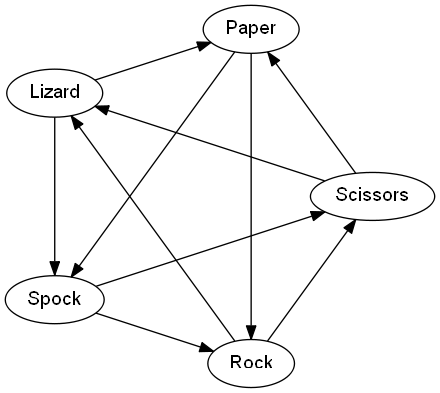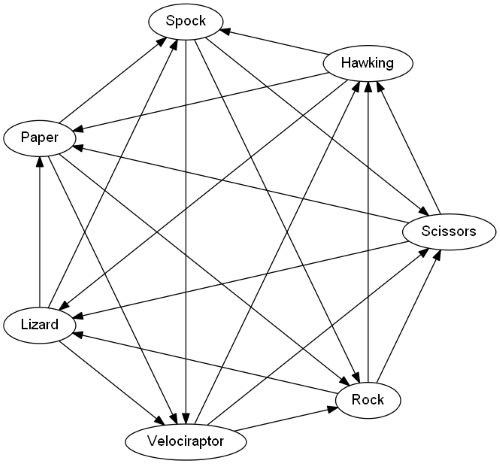# How Many Ways to Design Rock, Paper, Scissors, Lizard, Spock?

### Ever tried playing this fun spin on an old classic? Probably. But, have you ever looked at the math behind it and coded out the game? Read on to see how!

· Big Data Zone · Tutorial
Save
3.91K Views

In The Big Bang Theory, Sheldon Cooper explains an extension of the game Rock, Paper, Scissors by introducing two more possibilities, Lizard and Spock. Sam Kass and Karen Bryla invented the game before it became widely known via the television show.

The diagram below summarizes the rules:## Alternative Rules

Imagine yourself in the position of Sam Kass and Karen Bryla designing the game. You first try adding one extra move, but it turns out that's not possible.

The main property of Rock, Paper, Scissors is that no player has a winning strategy. That implies you can only add an even number of extra moves, keeping the total number of moves odd. That way, for every move one player makes, the other player has an equal number of winning and losing moves. Otherwise, some moves would be better and others worse. So you can't add just one move, say Lizard. You have to add two (or four, or six, ...).

How many ways could you assign rules to an extension of Rock, Paper, Scissors adding two more moves?

Number the possible moves 1 through 5. We will make a table of which moves beat which, with +1 in the (i, k) position if move i beats move j and -1 if j beats i. There will be zeros on the diagonal since it's a tie if both players make the same move.

Let's start with the original Rock, Paper, Scissors. In order for this game to not have a winning strategy, the table must be filled in as below, with the only option being to set a = 1 or a = -1.

``````|---+----+----+----|
|   |  1 |  2 |  3 |
|---+----+----+----|
| 1 |  0 |  a | -a |
|---+----+----+----|
| 2 | -a |  0 |  a |
|---+----+----+----|
| 3 |  a | -a |  0 |
|---+----+----+----|``````

If 1, 2, and 3 correspond to Rock, Paper, and Scissors, then a = 1 according to the usual rules, but we'll allow the possibility that the usual rules are reversed (if you don't like that, just set a = 1).

Next, we fill in the rest of the moves. The table must be skew-symmetric, i.e. the (i, j) element must be the negative of the ( j, i) element, because if (i, j) is a winning move then ( j, i) is a losing move and vice versa. Also, the rows and columns must sum to zero. Together these requirements greatly reduce the number of possibilities.

``````|---+----+----+----+----+----|
|   |  1 |  2 |  3 |  4 |  5 |
|---+----+----+----+----+----|
| 1 |  0 |  a | -a |  b | -b |
|---+----+----+----+----+----|
| 2 | -a |  0 |  a |  c | -c |
|---+----+----+----+----+----|
| 3 |  a | -a |  0 |  d | -d |
|---+----+----+----+----+----|
| 4 | -b | -c | -d |  0 |  d |
|---+----+----+----+----+----|
| 5 |  b |  c |  d | -d |  0 |
|---+----+----+----+----+----|``````

The values of a, b, and c may each be chosen independently to be 1 or -1. If b + c = 0, then d can be chosen freely. Otherwise b and c have the same sign, and d must have the opposite sign. So all together there are 12 possibilities (6 if you insist a = 1). These are listed below.

``````|---+---+---+---|
| a | b | c | d |
|---+---+---+---|
| + | + | + | - |
| + | + | - | + |
| + | + | - | - |
| + | - | + | + |
| + | - | + | - |
| + | - | - | + |
| - | + | + | - |
| - | + | - | + |
| - | + | - | - |
| - | - | + | + |
| - | - | + | - |
| - | - | - | + |
|---+---+---+---|``````

The version of the rules used on The Big Bang Theory corresponds to the second row of the table above: a = b = d = 1 and c = -1.

## Simpler Solution

Here's another way to count the number of possible designs. Suppose we start with traditional Rock, Paper, Scissors, corresponding to a = 1 in the notation above. Now let's add the rules for Lizard. We can pick any two things from {Rock, Paper, Scissors, Spock} and say that Lizard beats them, and then the other two things must beat Lizard. There are 6 ways to choose 2 things from a set of 4.

Once we've decided the rules for Lizard, we have no choice regarding Spock. Spock's rules must be the opposite of Lizard's rules in order to balance everything out. If we decide Lizard beats Rock, for example, then Rock must beat Spock so two things beat Rock and Rock beats two things.

If we're willing to consider reversing the usual rules of Rock, Paper, Scissors, i.e. setting a = -1, then there are 6 more possibilities, for a total of 12.

By the way, we can see from the approach above how to add more moves. If we wanted to add Stephen Hawking and Velociraptor to our game, then we have 20 choices: we choose 3 things out of 6 for Hawking to beat, and the rest of the rules are determined by these choices. Velociraptor has to be the anti-Hawking. If we decide that Hawking beats Paper, Lizard, and Spock, then we'd get the rules in the diagram below.## Fair Subsets

You might want to design the game so that for any subset of three moves you have a game with no winning strategy. Here's an example as to why. If the subset (1 , 2, 4) is a fair game, then a = c. But if the subset (2, 3, 4) is a fair game, then a = -c. So one of the two games must have a winning strategy.

## Graphing the Rules

The first graph above was made with GraphVis using the code below.

``````digraph rock {
node [fontname = "helvetica"]

"Rock"     -> "Scissors"
"Rock"     -> "Lizard"
"Paper"    -> "Rock"
"Paper"    -> "Spock"
"Scissors" -> "Paper"
"Scissors" -> "Lizard"
"Lizard"   -> "Spock"
"Lizard"   -> "Paper"
"Spock"    -> "Rock"
"Spock"    -> "Scissors"

layout = circo
}``````

Save the code to a file, say `rock.gv`, then run the command

``dot -Tpng rock.gv > rock.png``

to produce a PNG file.

Topics:
combinatorics, big data, graphviz, data visualization

Published at DZone with permission of John Cook, DZone MVB.

Opinions expressed by DZone contributors are their own.